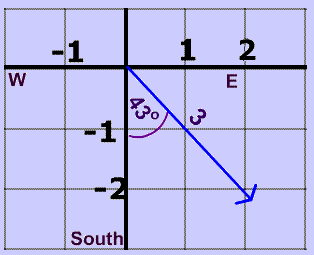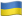# What Is Magnitude In Physics: Everything You Need To Know

In physics and mathematics, one of the most important topics is vectors. A vector is a quantity that has two crucial components: magnitude and direction. For example, 20 Newton cannot be used as a full description of a force that is acting on an object. The complete description of such a force would be something like 10 Newton, downward (captures both magnitude and direction).In this post, we are going to delve deeper into the concept of magnitude to answer the question: “How do you calculate the magnitude of a vector?” We will also use examples to demonstrate how you can calculate the magnitude of different problems.

## Magnitude In Physics: Understanding Resultant Vector

Before you can effectively calculate the magnitude of any force, the first step is to understand vectors. If you look at ‘scalars’ such as temperature or speed, they have a value that demonstrates everything about a specific aspect. Take the example of a scaler like 20 miles per hour. That will tell you how fast an object is moving. However, vectors are different.

A vector differs from common scalers because it has both magnitude and direction. In weather reports, you can easily tell how fast the wind was moving and in what direction.

When looking at magnitude definition physics, vectors are split into several components. In physics, the vectors are represented using X and Y coordinates. See the description in the image below:### How To Find Magnitude Of Simple Numbers

What is magnitude in physics?

Magnitude helps to tell how big an impact is towards a specific direction.

Finding the magnitude of simple numbers is rather direct. If you take a number such as 1, 2, 4.5, or other simple numbers, the magnitude is the same number. If the number is -7, take the absolute value, the magnitude is 7.

Magnitude is the distance (call it mathematical distance) from zero. That implies that in the case of 7, or -7, the object is a distance of seven from zero. That is the only time in physics when you are allowed to ignore the negative. But you should not apply it elsewhere in physics or mathematics because negative signs are very important.

### How To Calculate The Magnitude Of A Vector (A Single Force)

If you have been thinking of how to find the magnitude of a force vector, you need to use the components together with Pythagoras theorem, creating a magnitude formula in physics. Here, you need to think of Y coordinate as the height of a triangle and X coordinate as the force. Then, the hypotenuse becomes the resultant force from X and Y. Here is a demonstration:

If a force, call it 4 Newtons (N) moving in X-direction and 3 N in Y-direction, the Pythagoras theorem can help you to calculate the magnitude. You can express it using the equation below: (with x for the x-coordinate and y for the y-coordinate).

F= √(x2 + y2)

To put it differently, the resultant force is the sum of x2 and y2. Here is how to work it out in your problems.

F = √(42 + 32) N

= √(16 + 9) N= √25 N = 5 N

Therefore, the magnitude of this force is 5 N.

At this point, one might ask: “What is the formula of finding the resultant vector when you have three component forces. You will still use the same equation but add another component, Z coordinate. This means you will have Y force, X forces, and Z coordinate. Therefore, here is the formula to use:

F=√(x2 + y2+ z2)

### The Resultant Magnitude Of Two Or More Vectors

If you have several forces acting on an object, you need first to calculate the resultant vector. That is pretty straightforward. The secret is ensuring that you add the corresponding X and Y components. Here is a demonstration that should help you solve your physics homework.

Think of a boat in the sea using the wind to sail. The water is impacting 4 N in an X-direction and 1 N in the Y-direction. Besides, wind adds another force of 5 N in the X-direction and 3 N in the Y-direction. Therefore, the resultant vector for the X-coordinate will be 4+5=9 N and for the Y-coordinate will be 3+1=4 N. This simply means that the 9 N will be acting in the X direction while 4 N will be exerted in the y-direction. Now, to calculate the magnitude of the resultant force, here is the equation to apply.

F= √(x2 + y2)

= √(92 + 42) N = 9.85 N

### Calculating Magnitude In Physics … Simple And Fun, Right?

Although some college students have often indicated that how to calculate magnitude using the parallelogram method is difficult, we have demonstrated that it is indeed simple. In reality, it is applied in almost every scenario you can think of such as driving to work, sailing in the sea, firing a gun, and operating machines. You only need to understand what is magnitude in physics and answer the question: “Does magnitude have a direction?” Then, follow the formulas provided in this post.

If you still find it difficult to grasp the meaning concept of the resultant of two vectors, magnitude and direction, and how to calculate magnitude, it is not the time to give up. There’s always the option of paying someone to do your homework, so make sure to seek writing help from good and cheap writing services, like ours. Our writing services have experts in physics who can help to do any calculation in vectors and magnitude. Then, keep practicing on how to find the resultant vector, and you will finally grasp the gist of doing all the calculations on vectors.

Let's stand with the heroesAs Putin continues killing civilians, bombing kindergartens, and threatening WWIII, Ukraine fights for the world's peaceful future.

Donate Directly to Ukraine